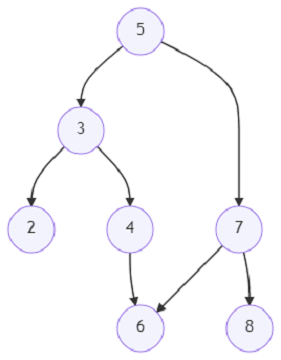# Program to fix a erroneous binary tree using Python

Suppose, we are given a binary tree that has a problem; one of the node's right child pointer points to another node at the same level in the binary tree erroneously. So, to fix this problem,we have to find out the node that has this error and delete that node and its descendants except the node that it is erroneously pointing to. We return the root node of the fixed binary tree.

So, if the input is likeWe can see that there is an erroneous link between 4 and 6. The right child pointer of 4 points to 6.

then the output, the inorder representation of the corrected tree will be − 2, 3, 5, 6, 7, 8,

Node 4 is deleted as it has an erroneous link to node 6.

To solve this, we will follow these steps −

• q := a new deque containing the root

• p := a new map

• visited := a new set

• while q is not empty, do

• cur := pop leftmost element of q

• if cur is present in visited, then

• is_left := p[p[cur, 0]]

• grand_p := p[p[cur, 0]]

• if is_left is not null, then

• left of grand_p := null

• otherwise,

• right of grand_p := null

• return root

• if left of cur is not null, then

• p[left of cur] := a new tuple (cur, 1)

• insert left of cur at the end of q

• if right of cur is not null, then

• p[right of cur] := a new tuple (cur, 0)

• insert right of cur at the end of q

• return root

Let us see the following implementation to get better understanding −

## Example

import collections
class TreeNode:
def __init__(self, data, left = None, right = None):
self.data = data
self.left = left
self.right = right
def insert(temp,data):
que = []
que.append(temp)
while (len(que)):
temp = que
que.pop(0)
if (not temp.left):
if data is not None:
temp.left = TreeNode(data)
else:
temp.left = TreeNode(0)
break
else:
que.append(temp.left)
if (not temp.right):
if data is not None:
temp.right = TreeNode(data)
else:
temp.right = TreeNode(0)
break
else:
que.append(temp.right)
def make_tree(elements):
Tree = TreeNode(elements)
for element in elements[1:]:
insert(Tree, element)
return Tree
def search_node(root, element):
if (root == None):
return None
if (root.data == element):
return root
res1 = search_node(root.left, element)
if res1:
return res1
res2 = search_node(root.right, element)
return res2
def print_tree(root):
if root is not None:
print_tree(root.left)
print(root.data, end = ', ')
print_tree(root.right)
def solve(root):
q = collections.deque([root])
p, visited = dict(), set()
while q:
cur = q.popleft()
if cur in visited:
grand_p, is_left = p[p[cur]]
if is_left: grand_p.left = None
else: grand_p.right = None
return root
if cur.left:
p[cur.left] = (cur, 1)
q.append(cur.left)
if cur.right:
p[cur.right] = (cur, 0)
q.append(cur.right)
return root
root = make_tree([5, 3, 7, 2, 4, 6, 8])
print_tree(solve(root))

## Input

root = make_tree([5, 3, 7, 2, 4, 6, 8])
link_from.right = link_to
2, 3, 5, 6, 7, 8,